# NCERT Solutions For Class 10 Maths Chapter 2 Exercise 2.1 Polynomials

Download NCERT Solutions For Class 10 Maths Chapter 2 Exercise 2.1 – Polynomials. This Exercise contain a question, for which detailed answers have been provided in this note. In case you are looking at studying the remaining Exercise for Class 10 for Maths NCERT solutions for other Chapters, you can click the link at the end of this Note.### NCERT Solutions For Class 10 Maths Chapter 2 Exercise 2.1 – Polynomials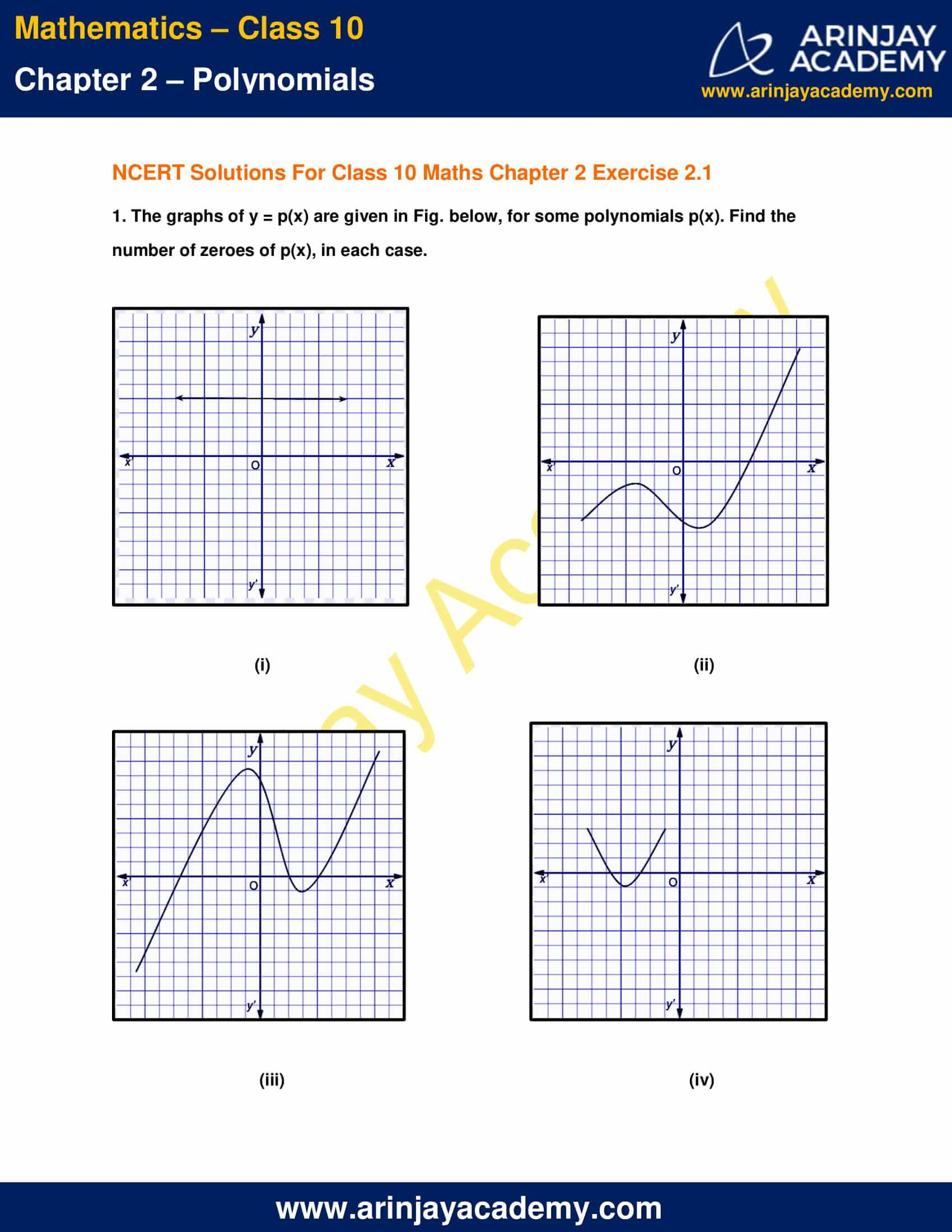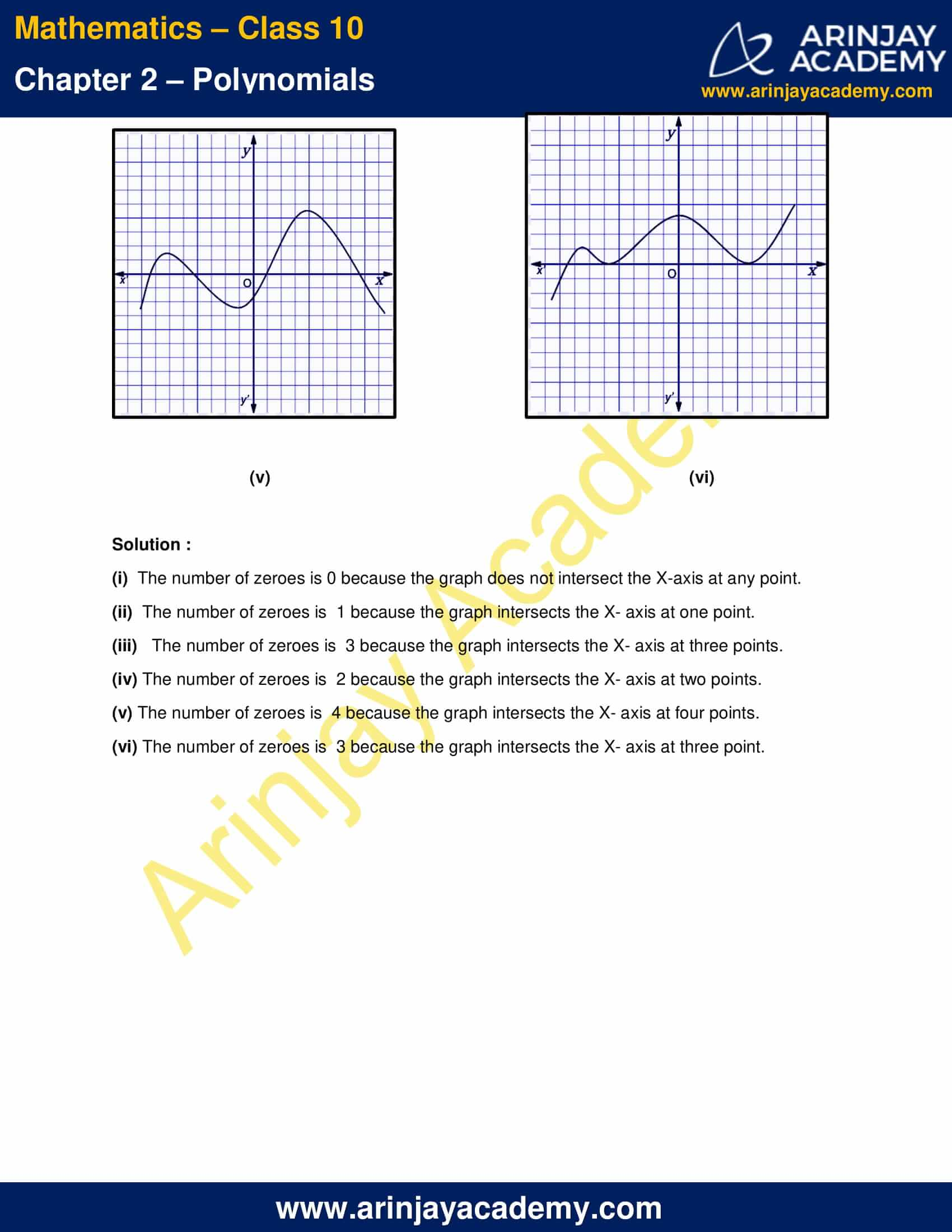NCERT Solutions For Class 10 Maths Chapter 2 Exercise 2.1 Polynomials

1. The graphs of y = p(x) are given in Fig below, for some polynomials p(x). Find the number of zeroes of p(x), in each case.

Solution :

(i)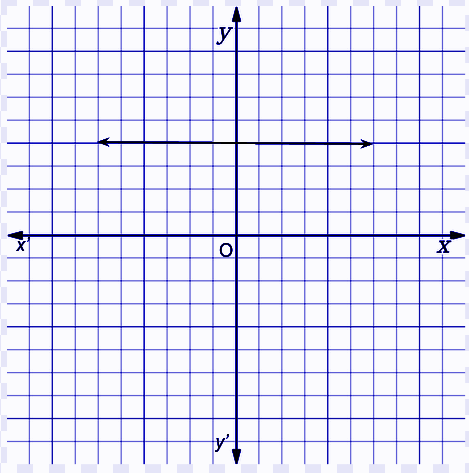The number of zeroes is 0 because the graph does not intersect the X-axis at any point.

(ii)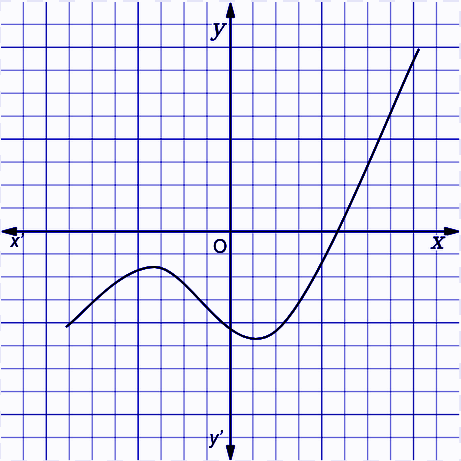The number of zeroes is  1 because the graph intersects the X- axis at one point.

(iii)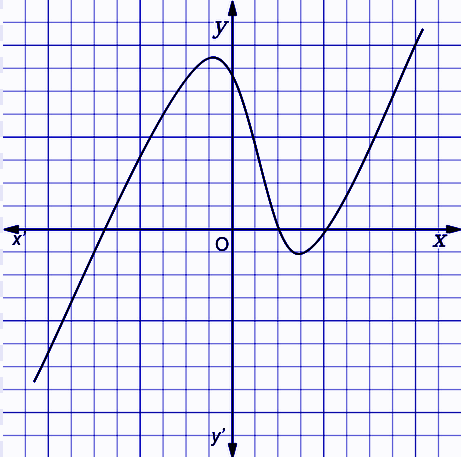The number of zeroes is  3 because the graph intersects the X- axis at three points.

(iv)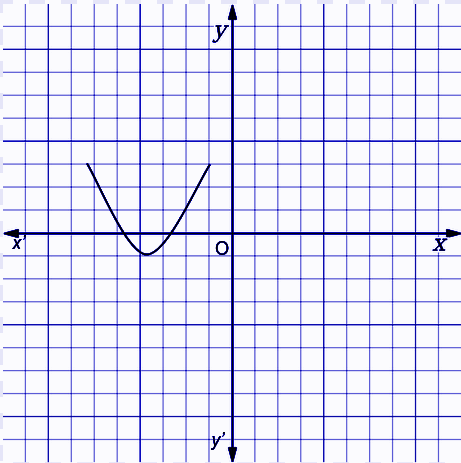The number of zeroes is  2 because the graph intersects the X- axis at two points.

(v)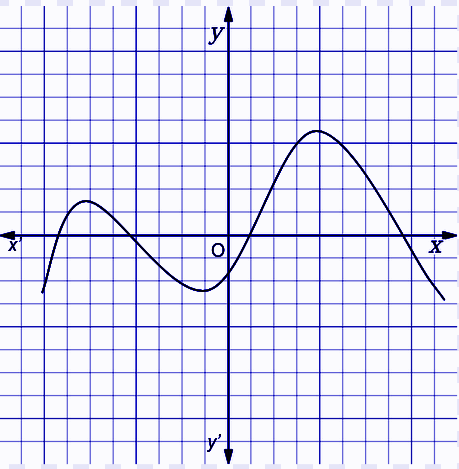The number of zeroes is  4 because the graph intersects the X- axis at four points.

(vi)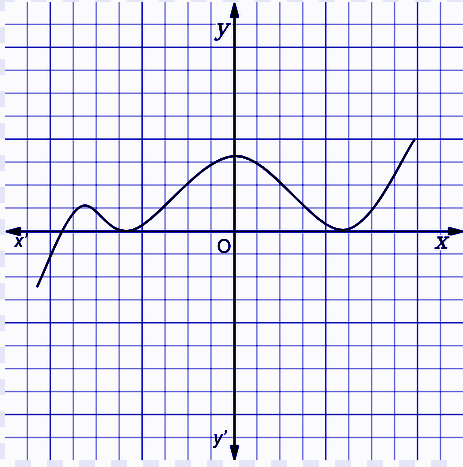The number of zeroes is  3 because the graph intersects the X- axis at three point.

NCERT Solutions for Class 10 Maths Chapter 2 Exercise 2.1 – Polynomials, has been designed by the NCERT to test the knowledge of the student on the topic – Geometrical Meaning of the Zeroes of a Polynomial